### Eyes of the beholderHover over the thumbnail for a full-size version.

Author im_bad_at_N author:im_bad_at_n ibannart n-art nart rated 2010-10-05 2010-10-05 5 by 12 people. \$Eyes of the beholder#im_bad_at_N#nart#00000000000000000000000000000000000000000000000000000000000000000000000000000000000000000000000000000000000000000000000000000000000000000000000000000000000000000000000000000000000000000000000000000000000000000000000000000000000000000000000000000000000000000000000000000000000000000000000000000000000000000000000000000000000000000000000000000000000000000000000000000000000000000000000000000000000000000000000000000000000000000000000000000000000000000000000000000000000000000000000000000000000000000000000000000000000000000000000000000000000000000000000000000000000000000000000000000000000000000000000000000000000000000000000000000000000000000000000000000000000000000000000000000000000000000000000000000000000000000|10^23,276!10^26,276!10^29,274!10^32,273!10^33,272!10^37,271!10^40,270!10^46,268!10^51,267!10^57,265!10^60,265!10^64,263!10^69,262!10^73,261!10^74,260!10^77,260!10^81,258!10^85,257!10^88,257!10^91,254!10^96,253!10^99,253!10^102,250!10^107,250!10^109,248!10^114,247!10^116,247!10^119,246!10^124,244!10^128,244!10^130,244!10^132,243!10^134,242!10^138,242!10^154,240!10^194,253!10^169,244!3^138,243!3^140,242!3^146,241!3^152,241!3^157,241!3^164,243!3^173,246!3^182,247!3^188,250!3^194,253!3^199,257!10^201,286!10^203,282!10^204,280!10^206,276!10^207,272!10^207,270!10^207,268!10^208,264!10^208,262!10^206,259!10^206,257!10^205,256!10^206,255!10^210,257!10^211,258!10^214,259!10^217,260!10^219,261!10^222,263!10^225,264!10^228,266!10^230,267!10^233,269!10^236,270!10^239,272!10^240,274!10^242,275!10^218,284!10^223,284!10^226,283!10^230,283!10^235,282!10^237,282!3^214,279!3^226,276!3^218,276!3^215,271!3^215,264!3^223,270!3^234,276!10^197,292!10^200,290!10^203,289!10^207,287!10^211,286!10^217,285!10^168,326!10^170,323!10^173,321!10^176,319!10^179,315!10^181,312!10^183,311!10^184,309!10^186,305!10^187,303!10^188,301!10^190,298!10^192,295!10^155,346!10^157,341!10^162,336!10^165,333!10^167,329!10^170,325!10^140,386!10^140,383!10^140,380!10^140,376!10^141,372!10^142,370!10^143,366!10^144,364!10^145,359!10^147,358!10^149,354!10^151,351!10^152,347!10^153,345!10^146,337!10^143,336!10^142,336!10^140,334!10^139,333!10^140,330!10^150,336!10^150,335!10^151,333!10^152,330!10^152,326!10^152,325!10^152,324!10^152,324!10^151,323!3^138,325!3^141,321!3^144,319!3^146,319!3^149,318!3^90,379!3^91,376!3^95,368!3^96,366!3^99,360!3^102,358!3^105,354!3^107,352!3^109,350!3^112,346!3^120,343!3^124,339!3^127,336!3^131,334!3^136,333!3^129,397!3^132,392!3^134,387!3^137,380!3^137,376!3^138,372!3^139,370!3^140,365!3^141,360!3^145,355!3^147,350!3^149,346!10^34,422!10^30,423!10^33,422!10^41,421!10^46,421!10^50,421!10^54,421!10^58,420!10^62,420!10^67,419!10^71,417!10^76,416!10^80,415!10^85,413!10^90,412!10^97,411!10^102,409!10^107,408!10^110,406!10^113,405!10^117,404!10^120,402!10^123,401!10^127,399!10^53,415!10^48,416!10^38,416!10^33,417!10^32,417!10^35,416!10^45,415!10^52,415!10^58,415!10^61,415!10^68,414!10^73,413!10^79,411!10^83,410!10^87,409!10^91,408!10^94,406!10^100,405!10^104,404!10^111,401!10^115,399!10^121,397!10^124,394!10^127,392!10^128,391!10^128,389!10^127,386!10^127,386!10^125,386!10^121,389!10^115,393!10^110,395!10^104,398!10^98,400!10^92,401!10^87,403!10^81,404!10^76,406!10^69,408!10^61,411!10^54,412!10^45,413!10^33,414!10^28,414!10^23,416!10^24,421!10^29,407!10^35,408!10^41,410!10^46,409!10^54,407!10^61,406!10^67,406!10^71,405!10^77,402!10^80,401!10^87,398!10^93,395!10^98,394!10^103,391!10^109,389!10^114,386!10^120,383!10^126,381!10^129,380!10^99,388!10^102,386!3^105,384!10^108,383!3^111,381!3^113,379!10^115,377!3^118,376!10^122,374!10^125,373!3^129,372!10^133,371!3^102,378!3^109,374!3^115,367!3^119,363!3^124,360!3^131,356!3^120,369!3^127,365!3^133,363!3^139,352!10^28,404!3^24,401!3^33,401!10^36,401!3^41,402!10^44,402!3^49,402!10^53,402!10^59,402!10^64,400!3^70,398!3^77,397!10^81,395!3^85,394!10^90,390!10^95,389!3^25,390!3^32,391!3^37,392!3^45,393!3^51,393!3^60,393!3^67,391!3^71,391!3^75,390!3^79,389!3^30,382!3^41,382!3^54,384!3^62,382!3^68,381!3^76,380!3^27,370!3^34,372!3^46,374!3^57,373!3^70,371!3^79,369!3^34,360!3^48,359!3^64,360!3^74,357!3^85,355!3^91,346!3^78,341!3^63,343!3^38,343!3^41,333!3^52,317!3^75,310!3^56,330!3^35,319!3^68,323!3^86,324!3^102,307!3^98,332!3^108,319!3^124,310!10^25,487!10^27,486!10^32,483!10^35,481!10^40,477!10^44,473!10^46,471!10^51,467!10^54,463!10^56,461!10^61,456!10^67,451!10^73,446!10^77,441!10^81,439!10^86,436!10^91,433!10^95,430!10^104,426!10^106,424!10^112,421!10^117,419!10^121,415!10^125,412!10^129,408!10^132,404!10^135,401!10^138,398!10^27,501!10^35,494!10^45,484!10^53,477!10^58,472!10^65,466!10^70,461!10^78,455!10^85,449!10^93,445!10^46,495!10^59,485!10^65,479!10^75,473!10^85,464!10^94,457!10^100,452!10^108,448!10^103,464!10^109,460!10^115,455!10^113,472!10^119,466!10^123,481!10^115,488!10^93,472!10^85,479!3^104,437!3^113,434!3^122,426!3^129,421!3^118,446!3^128,439!3^134,433!3^147,425!3^124,455!3^135,449!3^141,442!3^150,436!3^156,417!3^167,412!3^173,405!10^140,413!10^151,407!10^158,402!10^167,396!10^171,391!10^143,397!10^144,395!10^147,393!10^152,389!10^156,386!10^159,383!10^162,379!10^164,377!10^168,374!10^173,371!10^177,368!10^180,364!10^183,361!10^186,357!10^189,354!10^191,351!10^193,349!10^197,346!10^199,343!10^201,341!10^204,338!10^160,395!10^169,387!10^177,379!10^185,373!10^193,365!10^201,357!10^207,347!3^180,393!3^190,381!3^200,374!3^207,366!3^211,357!3^161,426!3^172,418!3^182,410!3^187,399!3^194,392!3^203,384!3^212,377!3^220,367!3^154,444!3^166,437!3^175,427!3^183,419!3^190,412!3^170,445!3^178,438!3^186,431!10^55,575!10^60,571!10^63,569!10^67,566!10^71,563!10^74,560!10^79,557!10^84,554!10^91,551!10^94,549!10^99,547!10^102,545!10^106,543!10^109,542!10^117,538!10^122,534!10^126,531!10^129,528!10^132,524!10^136,521!10^138,519!10^141,516!10^143,513!10^146,511!10^151,508!10^157,502!10^159,499!10^160,496!10^164,492!10^167,489!10^170,487!10^174,484!10^177,481!10^181,478!10^186,475!10^190,471!10^193,468!10^198,465!10^202,461!10^208,456!10^215,450!10^218,447!10^221,445!10^224,442!10^227,437!10^230,433!10^231,429!10^233,426!10^236,424!10^238,421!10^241,418!10^242,415!10^245,412!10^125,489!10^73,485!10^105,475!10^127,498!10^130,510!3^128,466!3^138,459!3^132,479!3^145,469!3^147,455!3^138,486!3^138,500!3^149,493!3^161,449!3^155,463!3^221,349!3^227,340!3^233,329!3^214,340!10^214,340!10^220,332!10^225,328!10^230,321!10^234,310!10^236,302!10^205,333!10^208,329!10^213,324!10^217,319!10^219,316!10^222,314!10^226,310!10^227,307!10^230,303!10^232,299!10^236,293!10^238,289!10^241,286!10^243,282!10^245,279!10^247,277!10^249,273!10^252,268!10^256,263!10^259,260!10^263,256!10^264,254!10^268,249!10^273,246!10^276,244!10^281,240!10^282,239!10^283,238!10^287,235!10^290,232!10^294,229!10^298,227!10^301,225!10^305,222!10^311,218!10^315,216!10^321,212!10^324,211!10^327,209!10^328,208!10^331,207!10^335,205!10^337,204!10^342,204!10^345,202!10^347,201!10^351,200!10^356,199!10^361,199!10^365,200!10^370,201!10^373,201!10^378,203!10^381,203!10^383,203!10^388,204!10^393,205!10^398,207!10^402,208!10^406,209!10^412,212!10^417,215!10^422,216!10^427,216!10^433,217!10^437,217!10^443,218!10^448,218!10^454,217!10^456,217!10^458,217!10^459,216!10^247,410!10^249,409!10^252,407!10^257,404!10^258,402!10^260,401!10^264,396!10^268,392!10^270,390!10^275,387!10^277,384!10^280,382!10^284,379!10^286,376!10^288,372!10^289,370!10^291,367!10^293,365!10^296,363!10^299,360!10^302,358!10^304,355!10^306,353!10^308,351!10^310,349!10^312,347!10^314,346!10^316,344!10^322,341!10^323,341!10^319,336!10^317,334!10^316,333!10^321,338!10^324,340!10^326,342!10^330,347!10^332,349!10^334,350!10^336,352!10^339,357!10^342,361!10^343,363!10^344,364!10^347,368!10^348,371!10^349,374!10^351,376!10^342,368!10^340,365!10^338,363!10^336,363!10^333,362!10^330,361!10^326,360!10^323,360!10^321,361!10^320,362!10^310,364!10^286,404!10^287,400!10^287,400!10^288,397!10^289,393!10^290,390!10^290,388!10^292,384!10^294,380!10^295,378!10^295,377!10^297,374!10^299,372!10^301,370!10^303,368!10^308,364!10^313,361!10^322,357!10^307,359!10^311,355!10^316,352!10^318,349!10^322,344!10^324,344!10^329,351!10^333,354!10^334,356!10^329,354!10^322,352!10^314,352!10^310,355!10^315,354!10^325,348!10^287,375!10^291,372!10^297,366!10^303,361!10^293,376!10^295,372!10^298,370!10^301,367!10^304,365!10^310,361!10^313,361!10^318,361!10^325,360!10^291,420!10^289,419!10^287,417!10^285,416!10^284,414!10^283,411!10^282,409!10^281,407!10^281,406!10^281,403!10^293,423!10^295,425!10^298,426!10^302,427!10^306,427!10^308,427!10^312,427!10^316,427!10^319,425!10^323,424!10^327,422!10^331,421!10^335,420!10^339,419!10^342,418!10^345,418!10^348,417!10^351,415!10^356,413!10^360,412!10^362,410!10^363,410!10^366,409!10^369,407!10^371,406!10^375,405!10^377,404!10^380,403!10^383,402!10^387,400!10^390,400!10^392,398!10^359,526!10^361,523!10^363,520!10^365,518!10^367,515!10^368,512!10^369,507!10^371,501!10^372,498!10^372,494!10^373,490!10^374,486!10^376,479!10^376,474!10^376,471!10^377,467!10^377,462!10^378,458!10^379,454!10^380,450!10^381,446!10^381,443!10^382,441!10^383,436!10^384,432!10^385,427!10^385,421!10^387,417!10^388,411!10^390,406!10^391,403!10^392,400!10^393,396!10^395,392!10^397,388!10^398,385!10^400,383!10^401,380!10^403,376!10^406,371!10^409,365!10^410,362!10^413,358!10^414,355!10^416,352!10^418,347!10^421,344!10^422,342!10^424,339!10^428,332!10^430,328!10^432,325!10^435,321!10^437,317!10^439,314!10^442,310!10^443,308!10^444,303!10^447,297!10^451,294!10^452,290!10^454,287!10^456,283!10^457,282!10^456,279!10^457,277!10^459,273!10^461,271!10^462,265!10^464,262!10^466,260!10^467,257!10^469,255!10^377,482!10^284,418!10^282,421!10^282,425!10^282,427!10^282,431!10^283,436!10^283,441!10^283,445!10^284,450!10^284,453!10^285,457!10^286,460!10^287,466!10^287,468!10^287,471!10^290,475!10^291,478!10^293,481!10^295,485!10^296,488!10^297,490!10^300,493!10^302,496!10^303,497!10^304,499!10^306,502!10^308,504!10^311,508!10^314,511!10^318,513!10^321,515!10^325,516!10^328,519!10^331,521!10^334,523!10^339,526!10^343,527!10^347,528!10^351,529!10^353,528!10^358,528!10^359,529!10^361,528!10^287,428!10^290,430!10^294,433!10^301,434!10^287,439!10^295,442!10^300,441!10^290,451!10^296,452!10^303,451!10^290,458!10^294,461!10^301,460!10^292,466!10^300,467!10^307,466!10^296,474!10^299,474!10^305,472!10^297,479!10^302,478!10^311,475!10^304,488!10^310,486!10^316,484!10^312,498!10^316,497!10^321,492!10^321,504!10^324,501!10^327,498!10^328,513!10^330,509!10^334,505!10^341,522!10^342,519!10^345,513!10^354,518!10^354,518!10^355,515!10^358,509!3^307,438!3^313,439!3^321,436!3^325,434!3^331,433!3^341,430!3^346,427!3^351,425!3^358,423!3^365,419!3^371,416!3^377,413!3^382,410!3^306,445!3^313,445!3^321,444!3^328,443!3^336,441!3^343,439!3^350,436!3^358,433!3^373,424!3^380,420!3^305,457!3^310,454!3^318,453!3^325,450!3^330,449!3^338,448!3^353,438!3^359,435!3^373,425!3^357,506!3^360,501!3^363,497!3^366,491!3^368,481!3^370,476!3^372,468!3^373,462!3^375,454!3^376,447!3^377,438!3^347,503!3^358,486!3^365,460!3^366,458!3^337,499!3^340,492!3^352,468!3^359,447!3^328,489!3^330,484!3^335,477!3^345,461!3^320,477!3^322,474!3^331,468!3^319,462!3^326,460!10^158,506!10^169,503!10^160,505!10^166,505!10^171,507!10^178,507!10^182,507!10^188,508!10^193,508!10^197,507!10^201,507!10^185,484!10^187,488!10^192,492!10^194,494!10^197,497!10^200,501!10^202,503!10^207,505!10^210,506!10^213,507!10^215,507!10^216,508!10^219,509!10^220,509!10^223,507!10^226,508!10^228,509!10^232,511!10^237,513!10^241,514!10^245,515!10^249,517!10^258,517!10^262,516!10^266,514!10^268,514!10^272,512!10^276,512!10^280,511!10^286,511!10^292,511!10^296,511!10^301,512!10^305,512!10^309,512!10^188,483!10^193,487!3^194,486!3^197,487!3^203,491!3^207,493!3^210,497!3^214,499!3^222,499!3^226,497!3^232,494!3^237,495!3^241,497!3^250,501!3^255,504!3^259,505!3^264,506!3^267,508!3^271,508!3^273,508!3^277,506!3^283,506!3^293,506!3^302,508!3^262,499!3^249,495!3^241,494!3^237,493!3^229,495!3^220,497!3^212,498!3^208,498!3^203,496!3^198,492!3^193,481!3^200,487!3^207,492!3^212,494!3^219,496!3^225,494!3^232,494!3^241,493!3^249,495!3^256,498!3^262,500!3^270,504!3^277,505!10^281,397!10^282,393!10^284,389!10^286,387!10^289,382!10^284,381!10^291,373!10^294,371!3^301,411!3^295,404!3^296,393!3^302,383!3^309,377!3^318,372!3^291,410!3^307,418!3^318,415!3^310,407!3^303,398!3^306,389!3^315,382!3^323,378!3^314,395!3^326,406!3^339,400!3^324,394!3^351,398!3^333,388!3^314,384!10^271,402!10^270,413!10^270,432!10^272,449!10^276,466!10^279,478!10^286,490!10^296,499!10^281,495!10^277,484!10^268,471!10^265,455!10^262,440!10^262,427!10^263,411!10^261,453!10^257,440!10^257,432!10^369,527!10^372,527!10^375,528!10^379,529!10^382,531!10^386,532!10^390,534!10^394,535!10^395,535!10^398,536!10^400,537!10^404,538!10^407,539!10^411,540!10^416,542!10^419,543!10^423,545!10^426,546!10^429,547!10^431,548!10^433,549!10^438,549!10^441,550!10^448,551!10^453,552!10^458,552!10^460,552!10^465,551!10^468,551!10^471,550!10^476,549!10^479,548!10^484,545!10^487,543!10^489,542!10^492,541!10^493,540!10^497,537!10^503,534!10^506,531!10^509,527!10^514,523!10^516,519!10^519,516!10^522,512!10^523,510!10^526,506!10^528,503!10^529,501!10^531,498!10^533,494!10^535,491!10^537,487!10^539,483!10^541,480!10^542,477!10^544,473!10^545,470!10^546,466!10^547,463!10^425,542!10^423,540!10^421,532!10^420,526!10^420,520!10^421,516!10^422,510!10^436,539!10^430,530!10^429,521!10^431,511!10^435,498!10^440,484!10^446,471!10^448,543!10^440,525!10^441,510!10^447,496!10^453,479!3^460,463!3^454,461!3^465,479!3^456,490!3^451,505!3^447,523!3^455,535!3^463,544!3^470,541!3^481,536!3^462,533!3^473,529!3^458,518!3^470,520!3^485,508!3^497,519!3^462,511!3^473,503!3^486,491!3^461,500!3^443,531!3^433,533!3^440,515!3^444,501!3^447,490!3^453,476!3^472,489!3^472,467!3^455,450!3^464,437!3^464,455!3^469,442!3^486,525!3^503,511!3^490,502!3^474,481!10^476,554!10^477,555!10^480,555!10^482,555!10^484,555!10^487,555!10^490,555!10^493,554!10^497,554!10^469,553!10^465,554!10^476,555!10^489,556!10^494,556!10^498,555!10^503,554!10^508,554!10^514,554!10^522,554!10^528,553!10^532,551!10^535,549!10^539,546!10^541,544!10^544,541!10^547,537!10^548,534!10^549,530!10^550,527!10^551,523!10^553,519!10^554,513!10^554,507!10^554,503!10^553,499!10^552,496!10^552,489!10^550,484!10^550,479!10^550,474!10^549,471!10^548,465!10^548,462!10^548,460!10^483,550!10^486,550!10^489,549!10^496,548!10^502,548!10^510,547!10^516,544!10^527,544!10^531,543!3^505,542!3^516,538!3^525,536!3^534,532!3^538,528!3^540,523!3^541,515!3^544,508!3^544,500!3^521,526!3^528,521!3^534,513!3^535,505!3^540,495!3^543,488!3^534,536!10^535,540!10^539,537!10^542,533!10^518,546!10^526,545!10^535,541!10^370,520!10^375,519!10^418,492!10^422,485!10^426,478!10^430,471!10^432,465!3^425,479!3^429,464!3^431,459!3^434,451!3^382,500!3^392,495!3^403,493!3^385,490!3^395,486!3^405,478!3^414,475!3^402,466!3^411,456!3^425,452!10^422,505!10^422,498!10^424,495!10^380,295!10^383,295!10^388,291!10^393,287!10^397,285!10^401,282!10^408,284!3^403,282!3^407,290!3^412,294!3^415,302!3^417,306!3^436,291!3^431,283!3^429,276!3^427,269!3^425,263!3^422,257!3^419,249!3^352,256!3^354,248!3^356,241!3^354,233!3^357,253!3^359,244!3^363,249!3^457,229!3^458,233!3^459,243!3^462,249!3^460,255!3^453,248!3^450,241!3^448,235!3^447,227!3^432,223!3^438,227!3^443,233!3^446,242!3^448,251!3^449,257!3^449,265!3^449,270!3^451,277!3^429,223!3^419,220!3^415,219!3^410,215!3^400,212!3^369,207!3^378,209!3^390,214!3^400,216!3^447,227!3^451,226!3^458,223!3^458,237!3^463,247!3^468,347!3^473,354!3^477,357!3^483,361!3^489,358!3^449,346!3^459,348!3^463,338!3^460,333!3^457,328!3^455,324!3^455,321!3^452,313!3^448,306!3^446,302!3^437,292!10^443,305!10^445,303!10^448,299!10^460,203!10^461,207!10^461,213!10^461,216!10^461,221!10^462,224!10^463,229!10^465,232!10^467,240!10^469,245!10^470,246!10^472,253!10^473,257!10^474,260!10^476,262!10^478,267!10^481,271!10^484,276!10^486,279!10^488,284!10^488,289!10^490,293!10^491,297!10^494,301!10^495,305!10^495,307!10^498,311!10^499,313!10^502,314!10^506,315!10^509,317!10^513,318!10^515,319!10^519,319!10^523,319!10^526,319!10^529,319!10^533,319!10^533,318!10^535,316!10^538,315!10^541,312!10^544,311!10^548,309!10^550,308!10^554,305!10^558,303!10^562,300!10^567,298!10^570,296!10^574,294!10^579,291!10^581,289!10^589,285!10^595,280!10^599,277!10^603,275!10^606,271!10^503,271!10^507,274!10^512,275!10^516,277!10^520,277!10^525,277!10^528,277!10^532,276!10^535,276!10^537,276!10^543,272!10^546,271!10^499,261!10^503,262!10^509,266!10^513,267!10^516,268!10^519,268!10^523,268!10^526,268!10^533,268!10^537,268!10^540,268!10^549,269!10^553,267!10^557,267!10^519,188!10^520,192!10^520,200!10^520,204!10^519,212!10^518,217!10^517,221!10^515,229!10^514,234!10^516,242!10^521,244!10^509,230!3^529,240!3^538,235!3^525,241!10^516,180!10^516,176!10^513,171!10^511,167!10^472,147!10^477,148!10^480,149!10^483,152!10^486,156!10^490,158!10^492,159!10^496,161!10^499,161!10^505,162!10^475,166!10^477,166!10^482,164!10^485,163!10^489,161!10^477,173!10^478,177!10^480,180!3^483,183!3^487,186!3^492,186!3^500,186!3^507,185!3^512,185!10^497,175!10^494,174!10^492,172!10^492,170!10^494,168!10^497,168!10^499,169!10^501,173!10^499,174!10^496,174!10^494,170!10^495,170!10^498,168!10^499,168!10^495,171!10^493,171!10^491,170!10^494,168!10^498,168!3^460,202!3^460,201!3^461,197!3^461,192!3^461,189!3^462,186!10^469,146!10^469,147!10^468,152!10^467,156!10^466,160!10^465,164!10^465,171!10^465,173!10^465,177!10^463,181!10^463,185!10^462,188!10^462,191!10^462,194!10^461,197!10^461,200!10^460,205!10^460,207!10^461,210!10^462,213!10^466,225!10^469,229!10^472,231!10^478,234!10^481,236!3^490,235!3^496,234!3^474,221!3^484,226!3^466,210!3^473,203!3^482,216!3^480,247!3^472,241!3^481,257!10^571,245!10^581,250!10^587,248!10^592,247!10^597,244!10^603,236!10^609,232!10^615,225!3^559,235!3^569,239!3^578,237!3^591,234!3^597,228!3^582,241!3^598,249!3^604,247!3^610,241!10^606,267!10^607,264!10^611,258!10^612,255!10^614,252!10^615,249!10^617,244!10^618,239!10^619,235!10^620,230!10^621,226!10^621,224!10^622,220!10^623,214!10^623,210!10^624,206!10^625,202!10^625,197!10^590,100!10^592,103!10^595,106!10^598,110!10^600,114!10^601,119!10^603,123!10^604,126!10^606,130!10^607,130!10^608,134!10^610,139!10^611,143!10^612,145!10^616,150!10^616,153!10^617,157!10^619,161!10^621,165!10^622,169!10^622,175!10^623,180!10^623,182!10^624,185!10^624,187!10^625,190!3^607,220!3^616,214!10^549,169!10^553,169!10^558,168!10^566,165!10^578,161!10^579,161!10^587,161!10^594,164!10^598,166!10^601,167!10^595,177!10^589,177!10^584,176!10^578,175!10^571,174!10^567,174!10^593,183!10^589,186!3^584,189!3^574,191!3^570,189!3^567,188!3^561,185!3^551,185!3^558,182!3^563,180!10^572,177!10^570,178!10^571,179!10^578,183!10^579,180!10^574,178!10^569,178!10^575,183!10^578,181!10^578,180!10^575,172!10^578,173!10^583,173!10^589,175!10^530,84!10^530,84!10^533,82!10^535,80!10^538,77!10^540,76!10^545,74!10^549,74!10^552,74!10^556,75!10^561,76!10^563,78!10^566,79!10^570,82!10^574,86!10^578,89!10^582,92!10^585,95!10^587,98!10^590,99!10^592,101!10^483,88!10^481,89!10^484,87!10^490,85!10^495,83!10^502,82!10^507,81!10^512,81!10^516,82!10^524,83!10^526,84!10^529,86!10^479,92!10^478,97!10^478,103!10^478,108!10^477,113!10^476,117!10^475,122!10^474,127!10^473,131!10^473,135!10^473,137!10^470,140!3^610,205!3^615,198!3^616,187!3^618,178!3^616,168!3^479,124!3^485,114!3^489,106!3^496,93!3^486,95!3^484,105!3^480,132!3^492,111!3^503,89!3^579,103!3^584,114!3^588,126!3^590,134!3^592,145!3^602,155!3^609,157!3^595,150!3^603,140!3^599,132!3^594,124!3^591,117!3^587,109!3^576,94!10^548,41!10^553,43!10^558,46!10^570,52!10^574,53!10^579,56!10^583,60!10^590,65!10^596,70!10^601,74!10^605,77!10^607,82!10^610,87!10^614,91!10^619,96!10^625,101!10^629,107!10^633,112!10^637,117!10^641,124!10^645,128!10^650,137!10^654,144!10^659,151!10^661,156!10^667,167!10^672,174!10^676,181!10^680,185!10^684,190!10^669,198!10^664,189!10^660,181!10^657,177!10^653,173!10^649,171!10^646,168!10^642,166!10^638,161!10^633,156!10^625,147!10^621,141!10^619,134!10^614,122!10^611,113!3^655,163!3^650,155!3^644,147!3^638,144!3^634,138!3^630,127!3^627,121!3^622,115!3^617,111!3^611,105!3^608,101!3^605,98!3^601,94!3^597,91!3^594,90!3^590,86!3^587,83!3^583,80!3^576,77!3^571,75!3^566,74!10^535,82!10^530,77!10^524,72!10^523,70!10^523,65!10^543,75!10^539,72!10^535,68!10^534,65!10^555,72!10^550,69!10^549,66!10^564,71!10^561,69!3^569,63!3^580,68!3^589,72!3^595,76!3^603,82!3^612,89!3^614,90!3^618,93!3^622,97!3^651,31!3^655,34!3^650,25!3^655,32!3^659,42!3^662,48!3^664,55!3^666,61!3^668,66!3^671,75!3^674,84!3^678,95!3^680,102!3^683,109!3^565,38!3^569,40!3^575,43!3^581,46!3^587,48!3^597,53!3^603,58!3^607,60!3^613,64!3^622,70!3^627,72!3^632,76!3^639,80!3^643,83!3^648,86!3^652,89!3^657,95!3^661,99!3^665,105!3^669,109!3^673,113!3^677,118!3^681,123!10^592,63!10^598,66!10^602,68!10^609,72!10^613,75!10^619,78!10^625,81!10^631,84!10^635,88!10^640,91!10^645,95!10^650,99!10^654,104!10^659,110!10^665,116!10^669,120!10^672,125!10^678,131!3^605,78!3^610,79!3^616,83!3^623,89!3^630,95!3^634,99!3^638,102!3^643,107!3^646,110!3^650,114!3^653,117!3^656,121!3^659,125!3^663,130!3^669,134!3^674,138!3^679,143!3^681,146!3^687,153!3^691,158!3^695,164!3^697,166!3^698,169!3^701,173!3^587,35!3^593,37!3^598,40!3^604,44!3^613,49!3^620,53!3^624,55!3^628,57!3^635,62!3^639,66!3^643,70!3^647,75!3^652,78!3^657,82!3^662,87!3^667,91!3^671,94!3^675,100!3^605,29!3^610,32!3^618,35!3^623,40!3^627,42!3^631,45!3^639,50!3^643,53!3^648,57!3^653,61!3^658,67!3^661,72!3^664,75!3^667,80!3^670,85!3^673,91!3^676,95!3^681,104!3^682,110!3^684,116!3^687,124!3^689,128!3^690,132!3^693,138!3^695,144!3^697,149!3^700,153!3^701,156!3^703,161!3^705,165!3^635,30!3^638,32!3^643,36!3^646,40!3^650,45!3^654,51!3^658,57!3^663,64!3^668,72!3^671,78!3^675,83!3^706,169!3^705,171!10^685,135!10^686,139!10^689,144!10^691,149!10^694,154!10^695,158!10^697,164!10^700,168!10^702,172!10^704,176!10^705,183!10^707,190!10^709,196!10^712,202!10^714,210!10^717,218!10^720,225!10^721,227!10^722,229!10^725,234!10^729,241!10^730,246!10^734,254!10^737,262!10^739,267!10^742,273!10^744,278!10^745,284!10^749,295!10^755,303!10^757,306!10^761,310!10^765,315!10^768,319!10^770,322!10^745,288!10^683,161!10^685,162!10^690,169!10^693,174!10^696,178!10^697,185!10^698,192!10^701,202!10^704,208!10^707,213!10^709,219!10^711,225!10^715,230!10^719,236!10^721,244!10^723,249!10^724,256!10^727,263!10^729,267!10^731,275!10^734,282!10^735,287!10^741,298!10^741,301!10^747,309!10^750,314!10^754,320!10^757,324!10^761,327!10^765,330!10^767,333!10^664,145!10^668,147!10^671,151!10^674,156!10^678,162!10^681,168!10^685,175!10^688,180!10^691,185!10^698,194!10^701,200!10^703,205!10^706,212!10^708,218!10^710,223!10^626,160!10^627,161!10^633,166!10^638,171!10^643,176!10^646,178!10^650,183!10^653,186!10^656,192!10^659,199!10^662,206!10^665,212!10^670,219!10^675,225!10^629,174!10^633,177!10^638,180!10^644,185!10^646,189!10^649,194!10^653,200!10^655,206!10^658,210!10^661,215!10^665,224!10^665,231!10^667,239!10^669,245!10^670,246!10^630,182!10^625,181!10^632,186!10^636,190!10^640,195!10^643,200!10^646,205!10^650,214!10^652,224!10^654,232!10^654,237!10^653,243!10^650,249!10^647,262!10^643,266!10^638,271!10^631,277!10^629,280!10^626,284!10^621,288!10^614,292!10^605,296!10^600,297!10^596,298!10^592,299!10^646,263!10^647,260!10^650,256!10^651,222!10^649,215!10^647,209!10^597,290!10^602,288!10^607,287!10^612,283!10^617,278!10^621,274!10^625,269!10^629,265!10^633,262!10^637,256!10^642,249!10^645,245!10^646,241!10^647,236!10^647,229!10^646,225!10^645,220!10^642,213!10^639,208!10^636,204!10^633,199!10^631,195!10^629,211!10^632,216!10^633,220!10^634,226!10^634,232!10^632,237!10^630,242!10^630,244!10^628,247!10^624,254!10^621,256!10^618,260!10^627,227!10^639,228!10^637,237!10^634,247!3^689,202!3^692,208!3^695,214!3^700,222!3^703,231!3^705,237!3^707,245!3^710,252!3^713,259!3^715,265!3^716,270!3^717,275!3^719,281!3^721,286!3^725,295!3^730,303!3^733,307!3^737,313!3^740,318!3^743,325!3^747,331!3^753,338!3^758,345!3^762,348!3^766,350!3^671,255!3^675,268!3^677,273!3^680,281!3^681,287!3^684,292!3^687,299!3^690,305!3^693,310!3^697,315!3^701,320!3^706,326!3^708,330!3^712,336!3^717,342!3^720,345!3^727,350!3^734,354!3^740,358!3^745,361!3^751,364!3^757,367!3^764,369!3^767,370!3^771,371!3^678,235!3^681,243!3^683,249!3^683,256!3^683,263!3^685,266!3^687,272!3^690,279!3^691,285!3^694,291!3^699,298!3^704,306!3^708,311!3^714,317!3^718,323!3^721,326!3^726,330!3^730,334!3^735,338!3^738,341!3^744,345!3^748,349!3^752,352!3^757,354!3^761,357!10^671,202!10^672,208!10^674,211!10^676,217!10^679,223!10^682,229!10^685,236!10^689,243!10^693,249!10^697,253!3^682,210!3^685,217!3^689,225!3^693,232!3^696,245!3^700,253!3^703,262!3^708,272!3^712,282!3^716,290!3^721,298!3^724,305!3^730,315!3^733,321!3^741,330!3^695,262!3^701,274!3^703,280!3^707,287!3^712,296!3^716,303!3^718,309!3^723,317!3^727,323!3^732,330!3^736,334!3^739,337!3^659,242!3^659,246!3^659,250!3^660,257!3^661,262!3^663,270!3^663,275!3^665,282!3^667,286!3^671,293!3^675,300!3^679,309!3^683,315!3^687,321!3^691,325!3^696,331!3^701,337!3^705,341!3^710,347!3^717,352!3^720,355!3^728,363!3^734,368!3^741,373!3^743,375!3^753,379!3^759,382!3^764,383!3^767,384!3^734,377!3^739,385!3^740,388!3^745,395!3^752,399!3^761,406!3^765,408!3^751,386!3^759,394!3^766,400!3^698,488!3^701,493!3^705,498!3^707,502!3^711,506!3^716,508!3^721,509!3^729,514!3^734,511!10^731,434!10^734,443!10^737,453!10^738,459!10^740,463!10^743,469!10^745,474!10^747,479!10^750,486!10^752,491!10^754,496!10^756,500!10^757,504!10^760,509!10^764,512!10^766,515!10^771,519!10^687,576!10^687,573!10^685,569!10^684,565!10^668,452!10^666,458!10^667,468!10^669,474!10^670,478!10^670,486!10^673,492!10^674,500!10^676,507!10^678,520!10^680,527!10^681,533!10^683,542!10^684,548!10^686,558!10^687,562!10^681,514!10^679,512!3^719,429!3^722,440!3^723,453!3^727,464!3^732,474!3^735,481!3^708,440!3^712,460!3^716,472!3^722,481!3^701,448!3^707,471!3^717,485!3^721,489!3^729,448!3^732,461!3^734,470!3^738,478!3^741,484!3^737,491!3^731,493!3^739,497!3^745,495!3^731,573!3^734,569!3^737,564!3^746,557!3^760,549!3^743,557!3^751,552!3^758,547!3^750,571!3^757,566!3^766,562!3^765,570!3^747,574!3^749,563!3^762,552!3^691,568!3^696,561!3^701,553!3^708,546!3^715,543!3^710,568!3^714,556!3^718,552!3^700,574!3^692,549!3^736,507!3^743,505!3^749,502!3^752,500!3^755,497!3^759,493!3^763,488!3^764,485!3^765,479!3^765,472!3^765,469!3^765,459!3^765,456!3^765,447!3^765,445!3^707,393!3^719,398!3^726,402!3^731,404!3^736,408!3^745,415!3^749,420!3^754,426!3^759,433!3^764,440!3^720,409!3^739,417!3^750,422!3^757,428!3^765,436!3^742,406!3^753,413!3^759,421!3^762,427!3^728,508!3^664,507!3^666,505!3^675,498!3^676,495!3^680,491!3^682,489!3^683,485!3^684,481!3^686,475!3^687,470!3^689,459!3^689,456!3^690,448!3^690,442!3^672,357!3^682,360!3^690,364!3^698,370!3^703,374!3^707,381!3^708,391!3^706,398!3^702,402!3^696,406!3^684,409!3^678,412!3^669,415!3^677,327!3^681,334!3^683,345!3^684,353!3^684,368!3^685,380!3^686,391!3^687,401!3^687,407!3^687,417!3^687,428!3^687,441!3^691,438!3^696,433!3^704,425!3^714,413!3^720,403!3^676,332!3^693,336!3^684,335!3^690,343!3^696,346!3^710,360!3^715,364!3^724,369!3^693,355!3^703,363!3^713,373!3^723,382!3^728,388!3^690,419!3^697,414!3^703,406!3^715,390!3^691,391!3^697,385!3^698,380!3^652,267!3^653,272!3^653,283!3^653,291!3^652,299!3^653,308!3^653,313!3^654,321!3^653,330!3^652,336!3^651,345!3^649,354!3^648,365!3^647,372!3^646,378!3^644,386!3^644,390!3^643,397!3^642,405!3^640,416!3^640,418!3^643,436!3^643,439!3^640,432!3^640,426!3^644,441!3^642,448!3^642,457!3^643,464!3^646,471!3^646,481!3^647,488!3^646,495!3^648,502!3^650,507!3^653,513!3^655,515!3^657,521!10^658,526!10^660,531!10^662,536!10^664,541!10^668,547!10^671,554!10^673,558!10^675,562!3^656,540!3^654,532!3^650,523!3^647,515!3^642,504!3^641,495!3^638,487!3^635,479!3^635,473!3^634,468!3^633,452!3^633,445!3^633,439!3^632,434!3^632,426!3^632,418!3^632,410!3^632,400!3^632,394!3^633,388!3^633,382!3^635,373!3^636,367!3^637,356!3^637,352!3^641,343!3^641,335!3^642,331!3^644,322!3^645,312!3^646,306!3^647,298!3^647,290!10^647,284!10^648,279!10^648,276!10^646,283!10^641,293!10^635,304!10^633,312!10^631,321!3^639,283!3^637,288!3^630,294!3^626,295!3^618,298!3^610,302!3^604,304!3^598,306!3^589,309!3^588,311!3^588,311!3^580,319!3^578,324!3^574,331!3^571,337!3^566,344!3^564,350!3^562,356!3^560,363!3^557,369!3^555,376!3^554,382!3^553,390!3^553,396!3^552,410!3^551,417!3^550,425!3^551,431!3^552,440!3^553,444!3^554,455!3^557,462!3^560,469!3^562,476!3^566,487!3^567,491!3^569,496!3^574,508!3^576,514!3^580,525!3^583,533!3^586,540!3^591,553!3^593,561!3^597,566!3^602,573!3^598,532!3^593,525!3^590,519!3^587,512!3^584,505!3^582,499!3^582,498!3^577,486!3^576,481!3^575,478!3^572,472!3^569,466!3^569,462!3^567,455!3^566,449!3^562,438!3^562,432!3^562,426!3^562,420!3^562,417!3^562,411!3^563,405!3^563,403!3^564,397!3^565,386!3^567,377!3^568,374!3^570,367!3^572,362!3^575,353!3^577,345!3^579,340!3^585,330!3^588,324!3^592,317!3^594,312!3^597,309!3^614,305!3^614,309!3^613,314!3^612,325!3^613,331!3^612,341!3^613,347!3^614,353!3^617,362!3^618,372!3^620,381!3^621,390!3^621,400!3^623,408!3^623,417!3^624,424!3^623,434!3^623,441!3^624,449!3^628,462!3^628,469!3^628,480!3^627,487!3^622,503!3^623,513!3^623,519!3^623,531!3^626,539!3^627,545!3^627,551!3^619,520!3^615,510!3^613,506!3^612,499!3^609,493!3^609,488!3^608,477!3^608,466!3^609,461!3^609,452!3^610,446!3^612,441!3^616,428!3^616,424!3^626,364!3^626,352!3^627,343!3^628,334!3^629,328!3^630,325!3^613,367!3^612,372!3^609,378!3^605,389!3^601,398!3^599,407!3^597,415!3^593,426!3^589,436!3^588,445!3^587,458!3^589,467!3^590,476!3^598,485!3^599,483!3^598,475!3^597,462!3^598,448!3^599,441!3^600,437!3^603,428!3^606,422!3^607,416!3^610,405!3^613,402!3^614,398!3^579,445!3^579,435!3^580,426!3^581,422!3^581,413!3^583,405!3^585,396!3^587,389!3^588,382!3^591,375!3^593,369!3^596,363!3^598,358!3^599,353!3^601,344!3^603,336!3^604,327!3^605,321!3^606,312!3^604,315!3^603,321!3^599,331!3^594,339!3^590,346!3^585,356!3^582,365!3^578,374!3^575,384!3^574,390!3^573,399!3^572,407!3^570,421!3^571,427!3^574,435!3^663,294!3^663,297!3^664,307!3^664,317!3^665,327!3^663,337!3^662,344!3^661,350!3^659,360!3^658,375!3^656,386!3^654,399!3^654,404!3^654,412!3^653,422!3^652,430!3^652,436!3^651,449!3^651,456!3^652,469!3^654,480!3^657,487!3^674,351!3^673,361!3^672,375!3^671,385!3^672,392!3^673,400!3^674,410!3^672,418!3^670,428!3^671,436!3^672,443!3^671,449!3^670,453!3^528,363!3^530,361!3^533,354!3^538,348!3^544,342!3^547,338!3^551,333!3^504,371!3^505,366!3^510,358!3^511,356!3^534,360!3^540,358!10^479,86!10^479,83!10^479,75!10^481,74!10^482,70!10^483,66!10^490,80!10^490,75!10^490,68!10^492,64!10^499,78!10^499,74!10^502,70!10^512,76!10^513,70!10^515,67!3^599,30!3^592,23!3^631,28!3^615,21!10^374,524!10^381,522!10^385,521!10^393,518!10^398,515!10^402,512!10^410,506!10^412,503!10^416,498!10^366,526!10^384,513!3^392,506!3^402,501!3^379,511!10^107,449!10^115,456!10^122,468!10^126,483!10^128,496!10^132,514!10^727,569!10^732,562!10^740,556!10^746,551!10^753,545!10^761,540!10^767,536!10^563,49!10^472,67!10^479,66!3^251,422!3^247,431!3^244,441!3^250,459!3^257,473!3^265,488!3^259,477!3^255,486!3^244,476!3^242,461!3^235,444!3^252,446!3^262,470!3^259,421!3^267,423!3^267,459!3^270,473!3^273,482!3^272,492!3^304,229!3^312,226!3^325,224!3^334,221!3^342,216!3^348,212!3^358,210!3^347,220!3^355,217!3^367,216!3^381,218!3^394,220!3^371,218!3^391,221!3^406,226!3^415,228!3^422,230!3^428,232!3^433,235!3^440,240!3^394,222!3^469,270!3^473,279!3^475,284!3^477,287!3^480,293!3^483,300!3^487,309!3^464,281!3^465,290!3^472,297!3^477,303!3^480,307!3^486,315!3^492,318!3^498,322!3^503,323!3^508,325!3^514,326!3^519,326!3^533,325!3^525,326!3^539,323!3^547,317!3^554,316!3^561,316!3^568,315!3^566,307!3^571,307!3^576,308!3^582,298!3^586,297!3^285,364!3^290,357!3^295,350!3^282,370!3^280,374!3^299,345!3^303,338!3^306,332!3^311,341!10^311,325!10^316,328!10^319,332!10^320,333!10^307,324!3^328,371!3^341,379!3^309,318!3^315,320!3^322,324!3^328,328!3^333,333!3^338,338!3^343,345!3^347,352!3^354,361!3^412,487!3^424,466!3^449,395!3^461,397!3^472,404!3^449,383!3^465,390!3^474,394!3^450,385!3^464,402!3^471,408!3^436,383!3^474,405!3^485,408!3^482,397!3^494,400!3^496,408# Third Nart in a row, because they've just been sitting and collecting (electronic) dust in my files for too long. I'll post a link to the full view and thumb in the comments.

## Other maps by this author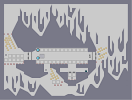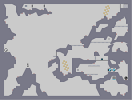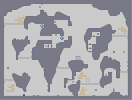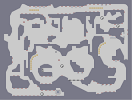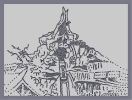Fuel for the fire Twice Around Cloud Cover Annoyance Splash Mountain Statue of Liberty

## Comments

Pages: (0)

### I have no clue

I made this a very long time ago. I'm glad you like it :)

Cool map*,

### Cool map

this is a cool , how long did it take for you to make this?

### :O

This Loaded?!!?!?!? Nice! :D

I delisted it :P

### I've seen this before

That or somebody has made an identical map in the same order of construction (you can tell by watching the map load.)

### ^^

can you make it next without clothes.

no just a joke, good n art^^

### Very nice man

Similar to mrgy's style, 4/5

### Hmm.. :P

Full [img440.imageshack.us]

### Links:Thumb: